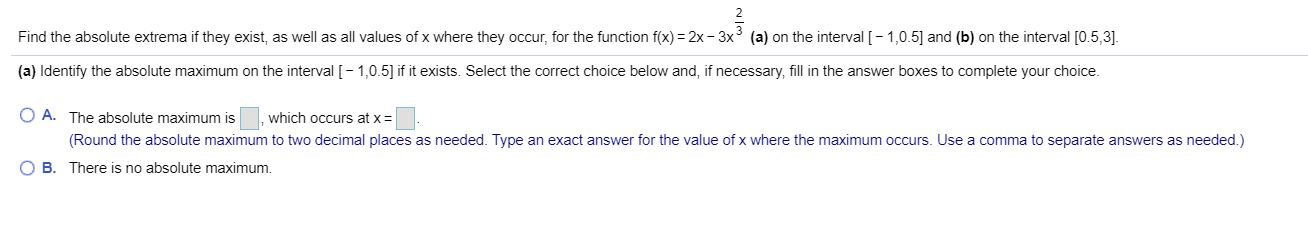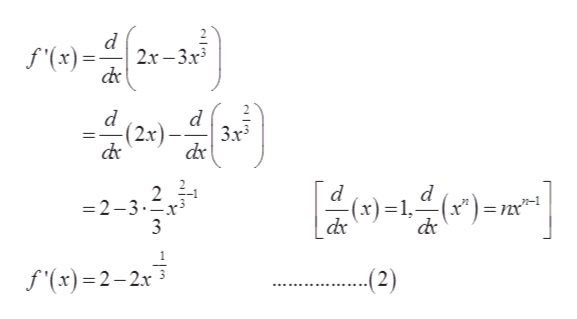# (a) on the interval [-1,0.5 and (b) on the interval [0.5,3]Find the absolute extrema if they exist, as well as all values of x where they occcur, for the function f(x) 2x -3y 3(a) Identify the absolute maximum on the interval [-1,0.5] if it exists. Select the correct choice below and, if necessary, fill in the answer boxes to complete your choiceO A. The absolute maximum iswhich occurs at x(Round the absolute maximum to two decimal places as needed. Type an exact answer for the value of x where the maximum occurs. Use a comma to separate answers as needed.)BThere is no absolute maximum.

Question
3 views

Find absolute maximum and absolute minimumhelp_outlineImage Transcriptionclose(a) on the interval [-1,0.5 and (b) on the interval [0.5,3] Find the absolute extrema if they exist, as well as all values of x where they occcur, for the function f(x) 2x -3y 3 (a) Identify the absolute maximum on the interval [-1,0.5] if it exists. Select the correct choice below and, if necessary, fill in the answer boxes to complete your choice O A. The absolute maximum is which occurs at x (Round the absolute maximum to two decimal places as needed. Type an exact answer for the value of x where the maximum occurs. Use a comma to separate answers as needed.) B There is no absolute maximum. fullscreen
check_circle

Step 1

Given,

Step 2

Take the derivative of equation (1),help_outlineImage Transcriptionclosed 2x-3x3 f'(x)= d d (2x) 3x3 2 =2-3 (x*)= (x)=1. 1 f'(x)2-2x (2) fullscreen
Step 3

For critical points, take deriv...

### Want to see the full answer?

See Solution

#### Want to see this answer and more?

Solutions are written by subject experts who are available 24/7. Questions are typically answered within 1 hour.*

See Solution
*Response times may vary by subject and question.
Tagged in

### Derivative